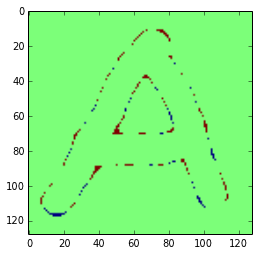This will follow Kevin Murphy's example in chapter 21 of Machine Learning: A Probabilistic Perspective, but we'll write the code in python with numpy and scipy. I wanted to understand the model better, so these are mostly notes for myself. Hope they are useful!

## Image Denoising¶

Let's assume we have a noisy, binary image, and we want to recover the original image. The code below will create our binary image coded as -1, 1 and the noisy version.

In :
import numpy as np
from scipy.signal import convolve2d
from scipy import stats
%pylab inline

Populating the interactive namespace from numpy and matplotlib

In :
img = imread('lettera.bmp')
arr = np.asarray(img, int)
mn = np.mean(arr)
arr[arr < mn] = -1
arr[arr >= mn] = 1
original_mean = np.mean(arr)
print original_mean

-0.477294921875

In :
sigma = 2
noisy_arr = arr + sigma*np.random.normal(size=arr.shape)

In :
imshow(arr)
title("Clean Image")

Out:
<matplotlib.text.Text at 0xa62090c>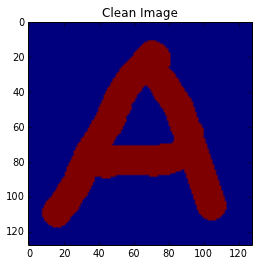In :
noisy_mean = np.mean(noisy_arr)
print noisy_mean
imshow(noisy_arr > noisy_mean)
title("Noisy Image")

-0.467782399643

Out:
<matplotlib.text.Text at 0xa7595cc>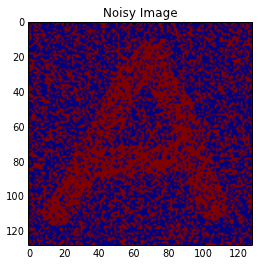## Simple Denoising¶

In :
# First, set up our averaging kernel
w_conv = np.ones((3, 3))
w_conv[1, 1] = 0

# The simplest denoising: just average nearby pixels.
less_noisy_arr = convolve2d(noisy_arr, w_conv, mode='same')  # mode='same' to keep same size
less_noisy_mean = mean(less_noisy_arr)
imshow(less_noisy_arr > less_noisy_mean)
title("Less Noisy Image by simple convolution")
figure()
num_iters = 20
for i in range(num_iters):
less_noisy_arr = convolve2d(less_noisy_arr, w_conv, mode='same')  # mode='same' to keep same size
less_noisy_mean = mean(less_noisy_arr)
imshow(less_noisy_arr > less_noisy_mean)
title("Less Noisy Image by repeated convolution.")
figure()
num_iters = 280
for i in range(num_iters):
less_noisy_arr = convolve2d(less_noisy_arr, w_conv, mode='same')  # mode='same' to keep same size
less_noisy_mean = mean(less_noisy_arr)
imshow(less_noisy_arr > less_noisy_mean)
title("Less Noisy Image by too many repeated convolutions.")

/home/guyrt/.virtualenvs/statistocat/local/lib/python2.7/site-packages/scipy/signal/signaltools.py:422: ComplexWarning: Casting complex values to real discards the imaginary part
return sigtools._convolve2d(in1, in2, 1, val, bval, fillvalue)

Out:
<matplotlib.text.Text at 0xaf8c4acc>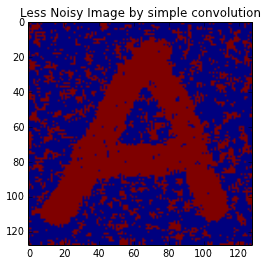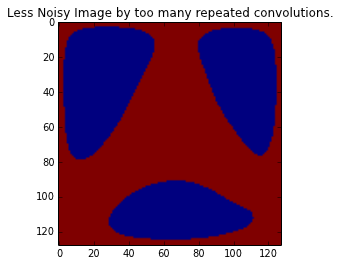While this averaging method might be easy to encode, it can be hard to tune. It also tends to "oversmooth". Eventually, it will oversmooth to a single, homogenous image!

## The Ising Model¶

How are we to recover the original image? The key insight is similar to our naive implementation: a pixel's value is usually correlated with its neighbors. Thus, we cast the problem as a Markov Random Field. The probability of an image y is

$$\log \tilde{p}(y) = - \sum_{s \neq t } y_s w_{st} y_t$$

There are a few observations to be made here. First, the weight $w_{st}$ describes the amount that we care about whether pixels $y_s$ and $y_t$ are equal to each other. If $w_{st} > 1$ then the probability of the total image is higher if $y_t$ and $y_s$ share the same value. This follows because $-1 * 1 * -1 = 1 * 1* 1 = 1$ but $1 * 1 * -1 = -1 * 1 * 1 = -1.$ It is often informative to think about Ising models in terms of a sum over products of pixel values encoded as -1, 1. Computationally, it's easier to treat them as a convolution, which we will see.

The values w_{s,t} should enforce our key insight: we only care about comparing $y_s$ and $y_t$ if they are near each other in the image. If that isn't true, then the weight is 0. We can recast the equation as

$$\log \tilde{p}(y) = \frac{1}{2} y^T W y$$

where W is a sparse block Toeplitz matrix. In keeping with our insight, we set $w_{st} = 1$ iff $y_s$ and $y_t$ are adjacent pixels. In fact, if we create a convolution matrix to simulate W, we see that the noise is somewhat reduced. Repeating the process does a fair job of removing noise, but it leads to "clumping": once enough pixels near each other take on a common value, they maintain that value rather well.

## Mean Field Update (Variational Inference)¶

However, the key insight above isn't enough to produce a solution.

Now, let's recover the original image using something a bit more powerful. To do this, we'll need to set up a bit of notation. Call the noisy image $y$ and the denoised image $x$, both of which are vectorized images (though our code uses convolve2, so we keep them as actual images). We want to find

$$argmax_x p(y, x) = p(x) p(y|x).$$

Theoretically, we want to find the image $x$ that is most likely to produce the noisy image $y$. If that feels backwards at first, that's okay. Identifying the best input given a know output is a very common machine learning technique.

The prior, $p(x)$ is constructed from the Ising model, so it encodes the idea that nearby pixels influence each other:

$$p(x) = \frac{1}{Z_0} exp(-E_0(x)) = \frac{1}{Z_0} exp(\sum_i\sum_{j \in nbhd(i)} W_{ij} x_i x_j).$$

Note: we don't need to know the value of our normalization constant $Z_0$. Below, we use the unnormalized probabilities $\tilde p = pZ$ for the constant terms in our equations.

In :
from scipy.sparse import dia_matrix, eye
def unnormalized_log_prior(x):
size = x.size
z = np.zeros(x.shape)
z[:3, :3] = 1
z[1, 1] = 0
z_vec = z.reshape(-1)
non_zeros = [e - x.shape - 1 for e, v in enumerate(z_vec[:x.shape * 3].reshape(-1)) if v > 0]
toep_mat = dia_matrix((size, size))
for non_zero in non_zeros:
toep_mat = toep_mat + eye(size, size, non_zero)
x_flat = x.reshape(-1)
print unnormalized_log_prior(2 * (noisy_arr > noisy_mean) - 1)
print unnormalized_log_prior(2 * (less_noisy_arr > less_noisy_mean) - 1)
print unnormalized_log_prior(ones(noisy_arr.shape))
print unnormalized_log_prior(ones(noisy_arr.shape) * -1)

13550.0
123030.0
130302.0
130302.0


The extrema of $p(x)$ occur when $x$ is a constant image, but those are probably not suitable solutions to the joint probability.

Now that we have a prior, we can examine the likelihood $p(y|x)$ of noisy image $y.$ $$p(y|x) = \prod_i p(y_i|x_i).$$ = \sum_i exp(-L_i(x_i)). $$for log likelihood loss function L_i. The loss function L_i gives us the probability of observing y_i given that the original image is x_i. Here, we assume that the distribution of y_i is normal with a mean determined by x_i. In our case, this is obviously true: we designed the noise function! That gives us a posterior: \begin{equation} p(x|y) = \frac{p(y|x) p(x)}{p(x, y)} = \frac{1}{Z} exp(\sum_i L_i(x_i) - E_0(x)) \end{equation} where E_0(x) is the Ising weight function defined above. Right away, we have a problem. The prior energy E_0 is defined by the Ising model, which includes interconnections between adjacent pixels in an image, which makes for a function that is difficult to optimize. (For more information on reasoning about Ising models in 2-d in physics, see Wikipedia.) This is where we use variational inference. Rather than deal with the likelihood, which is hard to optimize, we'll deal with a factored approximation. As a quick review, Variational Inference is an approximation technique for a probability distribution p. The idea is to create a class of distributions q that are easier to work with, and then to parameterize q to minimize the approximation error. In this case, our simplification is to assume that the posterior fully factorizes to$$ q(x) = \prod_i q_i(x_i, \mu_i) $$where parameter \mu_i is the mean value at pixel i. Note that (1) we can choose \mu_i to minimize q: it is a variational parameter, and (2) those means are independent of the surrounding pixels. That means we can derive the mean field update for each pixel independently. We start with$$ \log \tilde{p} (x_i) = x_i \sum_{j \in nbhd(i)} W_{ij} x_j + L_i(x_i) + const .$$For the mean field update, we need to compute (see Murphy section 21.3.1 for details)$$ \log q_j(x_j) = \mathbb E_{-q_j} [\log \tilde p(x)] + const $$and since E_{-q_j} (f) = \sum_{k \neq j} q(x_j, \mu_j | x_j) f(j) = \sum_{k \neq j} q(\mu_j) f(j),  we have$$ q_i(x_i) \propto exp(x_i \sum_{j \in nbhd(i)} W_{ij} \mu_j + L_i(x_i)) .$$That's the important theoretical step. Murphy derives an actual update using a great deal of exponential mathematical gymnastics. If you do read his derivation (page 738 in my copy), note that it uses L_i^+ \equiv L_i(+1) and L_i^- \equiv L_i(-1) which are the log likelihood functions centered at each of these two values. The variance in the likelihood controls the strength of the prior. This is the final update, which also incorporates a damping term:$$ \mu_i^t = (1 - \lambda)\mu_i + \lambda \tanh \big( x_i \sum_{j \in nbhd(i)} W_{ij} \mu_j + 0.5 (L_i^+ - L_i^-) \big) 

Damping is required because the mean field Ising update alone can lead to clumping around local extrema.

It's actually pretty easy to compute this in python.

In :
def unnormalized_log_prior2(x):
""" compute the log prior using adjacent pairs. """
size = x.size
z = np.zeros(x.shape)
z[:3, :3] = 1
z[1, 1] = 0
z_vec = z.reshape(-1)
non_zeros = [e - x.shape - 1 for e, v in enumerate(z_vec[:x.shape * 3].reshape(-1)) if v > 0]
toep_mat = dia_matrix((size, size))
for non_zero in non_zeros:
toep_mat = toep_mat + eye(size, size, non_zero)
x_flat = x.reshape(-1)

noisy_arr_copy = noisy_arr.copy()
lmbda = 0.5
for i in range(15):
logodds = log(stats.norm.pdf(noisy_arr_copy, loc=1, scale=2)) - log(stats.norm.pdf(noisy_arr_copy, loc=-1, scale=2))
noisy_arr_copy = (1 - lmbda) * noisy_arr_copy + lmbda * tanh(unnormalized_log_prior2(noisy_arr_copy).reshape(noisy_arr_copy.shape) + .5 * logodds)

In :
imshow(noisy_arr_copy)
print sum(np.abs(noisy_arr_copy - arr)) / arr.size

0.029547428632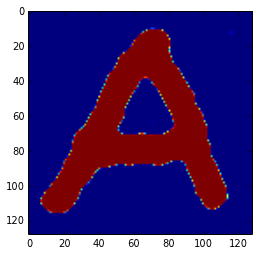In :
denoised_mu = np.mean(noisy_arr_copy)
noisy_arr_copy = 2 * (noisy_arr_copy > denoised_mu) - 1

In :
imshow(noisy_arr_copy)

Out:
<matplotlib.image.AxesImage at 0xb0992ac>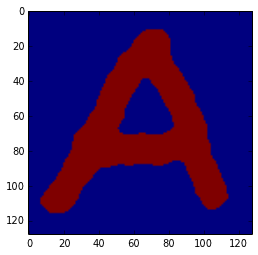In :
imshow(arr)

Out:
<matplotlib.image.AxesImage at 0xb4873ec>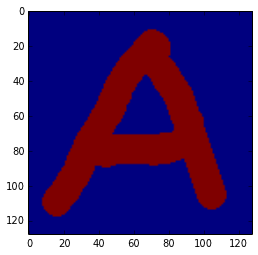In :
sum(np.abs(noisy_arr_copy - arr)) / arr.size

Out:
0

Here's where we experienced errors:

In :
imshow(noisy_arr_copy - arr)

Out:
<matplotlib.image.AxesImage at 0xb71df4c>